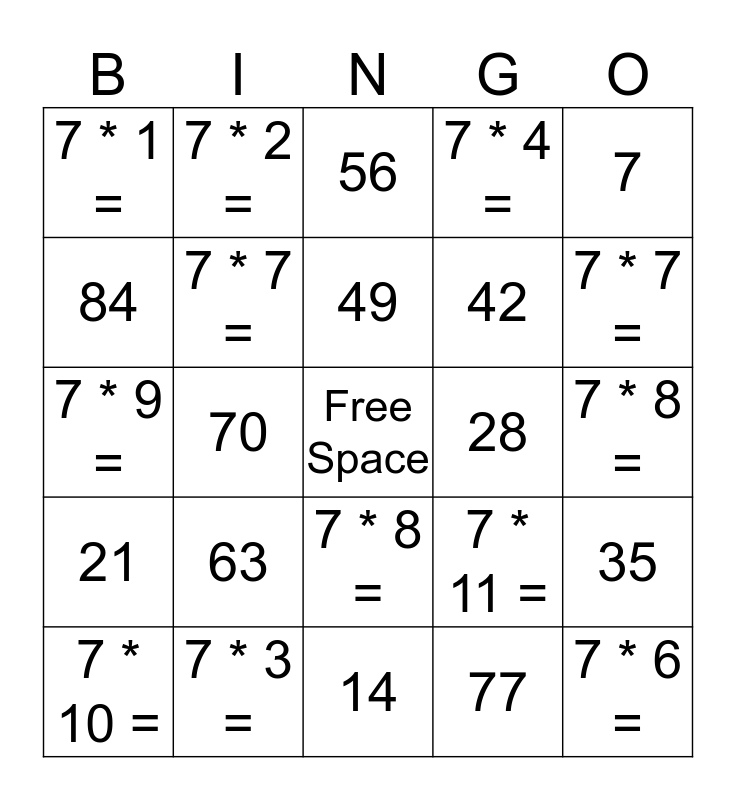# Multiplying by 7sThis bingo card has a free space and 24 words: 63, 7 * 3 =, 49, 7 * 10 =, 7, 7 * 1 =, 84, 7 * 2 =, 28, 7 * 8 =, 42, 7 * 9 =, 7 * 11 =, 35, 7 * 7 =, 77, 7 * 8 =, 56, 7 * 7 =, 70, 7 * 6 =, 21, 7 * 4 = and 14.

⚠ This card has duplicate items: 7 * 8 = (2), 7 * 7 = (2)

## Play Online